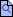Following toggle tip provides clarification

# How to Enter Math

Individual questions often contain specific instructions displayed directly with the question. When they don't, the following tables provide general guidelines for entering mathematical input.

## Basic Operations

OperationCommand
addition $(a+b)$a+b
subtraction $(a-b)$a-b
multiplication $(a \cdot b)$a*b
division $\left(\dfrac{a}{b}\right)$a/b
exponentiation $(a^{b})$a^b

## Constants & Symbols

Constant/SymbolCommand
$\pi$Pi
$\text{e}$exp(1)
$\text{i}$I
$\infty$infinity

## Functions

FunctionCommand
trigonometric $\left(\sin(x), \cos(x), \tan(x), \csc(x), \sec(x), \cot(x)\right)$sin(x), cos(x), tan(x), csc(x), sec(x), cot(x)
inverse trig $\big(\sin^{-1}(x)$ or $\arcsin(x)$, $\cos^{-1}(x)$ or $\arccos(x)$, $\tan^{-1}(x)$ or $\arctan(x)\big)$arcsin(x), arccos(x), arctan(x)
exponential $\left(e^{x}\right)$exp(x)
logarithmic $\left(\ln(x), \log_{a}(x)\right)$ln(x), ln(x)/ln(a)
square root $\left(\sqrt{x}\right)$sqrt(x)
$\text{n}^{th}$ root $\left(\sqrt[n]{x}\right)$surd(x,n)
absolute value $\left(\lvert x \rvert\right)$abs(x)
factorial $\left(n!\right)$factorial(n)

## Vectors & Matrices

FunctionCommand
horizontal vector $\begin{bmatrix}a & b & c\end{bmatrix}$<a|b|c>
vertical vector $\begin{bmatrix}a\\ b\\ c\end{bmatrix}$<a,b,c>
matrix $\begin{bmatrix}a & b & c\\ d & e & f\\ g & h & i\end{bmatrix}$<<a|b|c>, <d|e|f>, <g|h|i>>
OR
<<a,d,g> | <b,e,h> | <c,f,i>>

Always check your answers using the preview hyperlinkbefore submitting them. This can be found near the entry box of any formula questions.

Be sure to read each question carefully and make sure that you understand how to enter your answer.